The SIMILARITY Procedure

## Example 22.2 Similarity Analysis

This simple example illustrates how to compare two time sequences using similarity analysis. The following statements create an example data set that contains two time sequences of differing lengths.

```   data test;
input i y x;
datalines;
1   2  3
2   4  5
3   6  3
4   7  3
5   3  3
6   8  6
7   9  3
8   3  8
9  10  .
10 11  .
;
run;
```

The following statements perform similarity analysis on the example data set:

```   ods graphics on;
proc similarity data=test out=_null_
print=all plot=all;
input x;
target y / measure=absdev;
run;

```

The DATA=TEST option specifies that the input data set WORK.TEST is to be used in the analysis. The OUT=_NULL_ option specifies that no output time series data set is to be created. The PRINT=ALL and PLOTS=ALL options specify that all ODS tables and graphs are to be produced. The INPUT statement specifies that the input variable is X. The TARGET statement specifies that the target variable is Y and that the similarity measure is computed using absolute deviation (MEASURE=ABSDEV).

Output 22.2.1 Description Statistics of the Input Variable, x
The SIMILARITY Procedure

Time Series Descriptive Statistics
Variable x
Number of Observations 10
Number of Missing Observations 2
Minimum 3
Maximum 8
Mean 4.25
Standard Deviation 1.908627

Output 22.2.2 Plot of Input Variable, x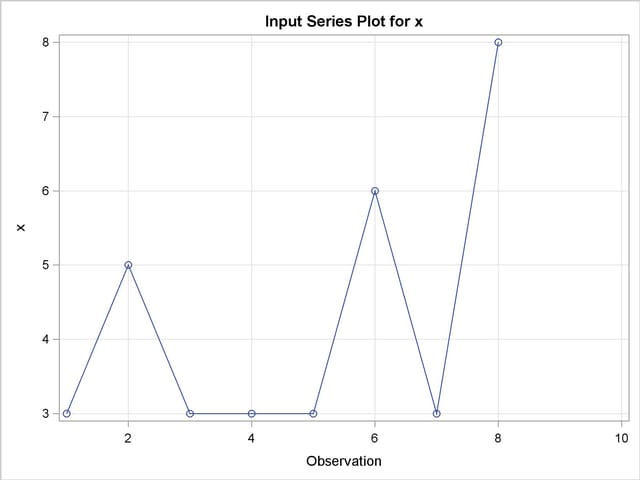Output 22.2.3 Target Sequence Plot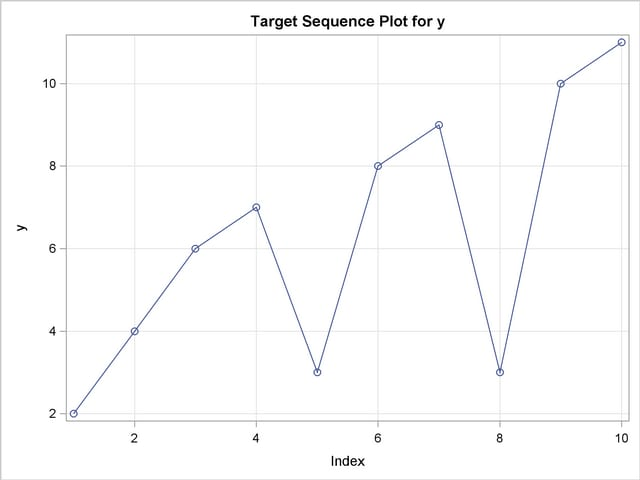Output 22.2.4 Sequence Plot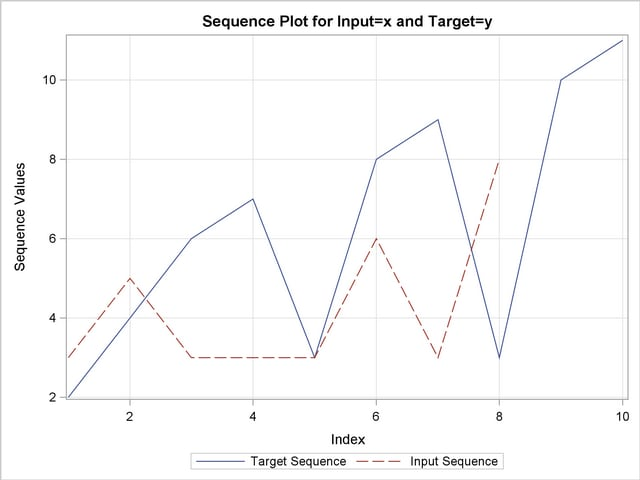Output 22.2.5 Path Plot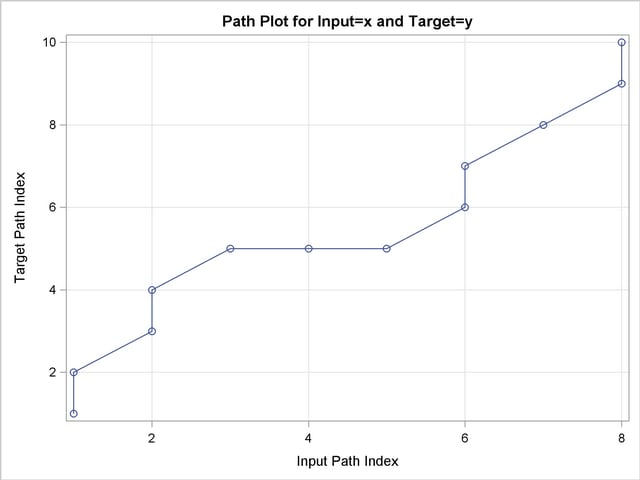Output 22.2.6 Path Sequences Plot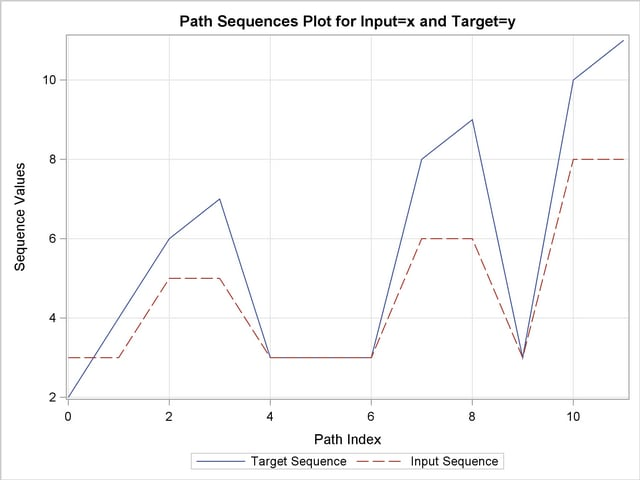Output 22.2.7 Path Sequences Scaled Plot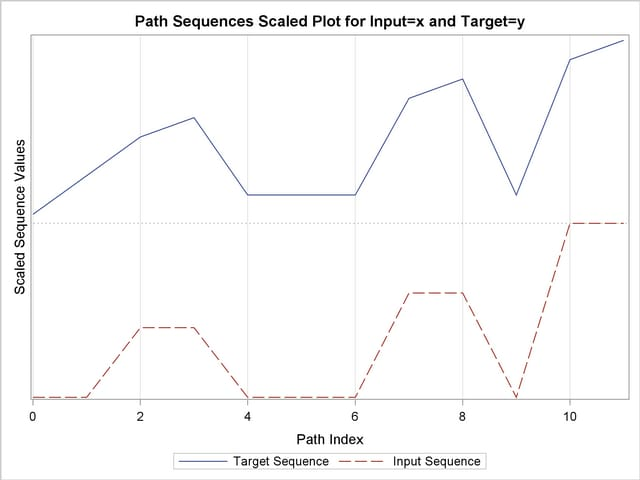Output 22.2.8 Path Distance Plot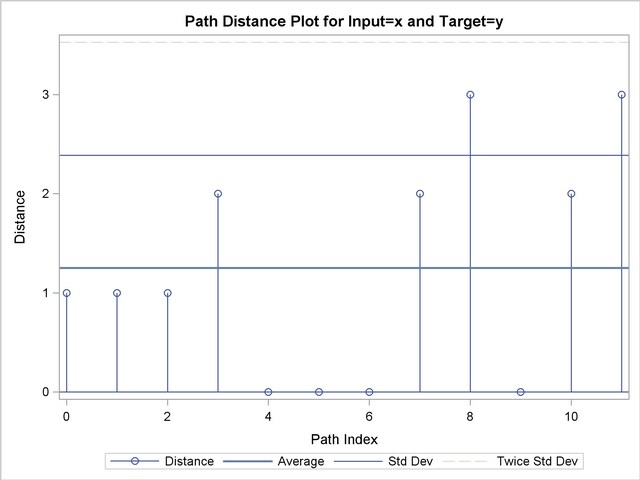Output 22.2.9 Path Distance Histogram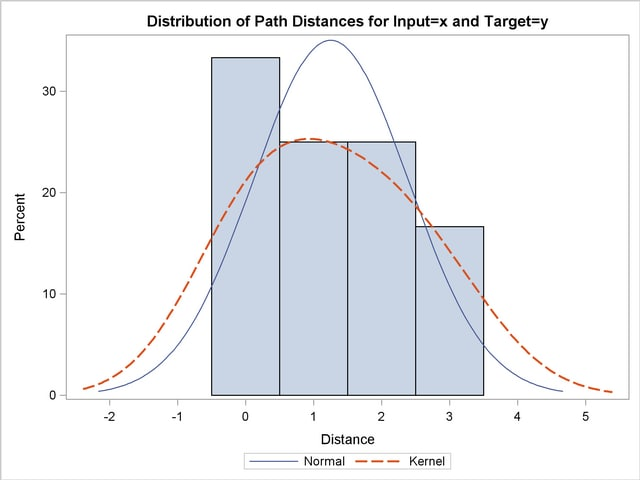Output 22.2.10 Path Relative Distance Plot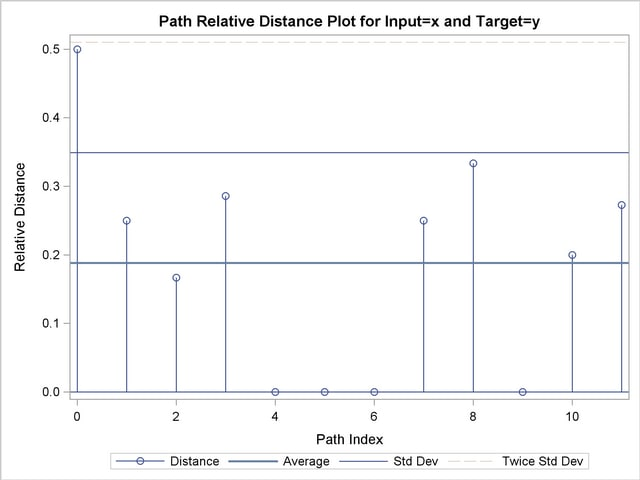Output 22.2.11 Path Relative Distance Histogram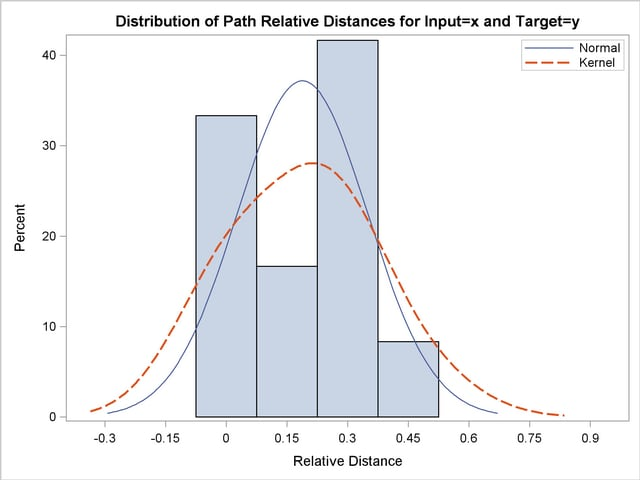Output 22.2.12 Path Limits
Path Limits
Limit Specified
Absolute
Specified Percentage Minimum Allowed Maximum Allowed Applied
Compression None None 2 9 9
Expansion None None 0 7 7

Output 22.2.13 Path Statistics
Path Statistics
Path Number Path Percent Input Percent Target Percent Maximum Path Maximum
Percent
Input Maximum
Percent
Target Maximum
Percent
Missing Map 0 0.000% 0.000% 0.000% 0 0.000% 0.000% 0.000%
Direct Maps 6 50.00% 75.00% 60.00% 2 16.67% 25.00% 20.00%
Compression 4 33.33% 50.00% 40.00% 1 8.333% 12.50% 10.00%
Expansion 2 16.67% 25.00% 20.00% 2 16.67% 25.00% 20.00%
Warps 6 50.00% 75.00% 60.00% 2 16.67% 25.00% 20.00%

Output 22.2.14 Cost Plot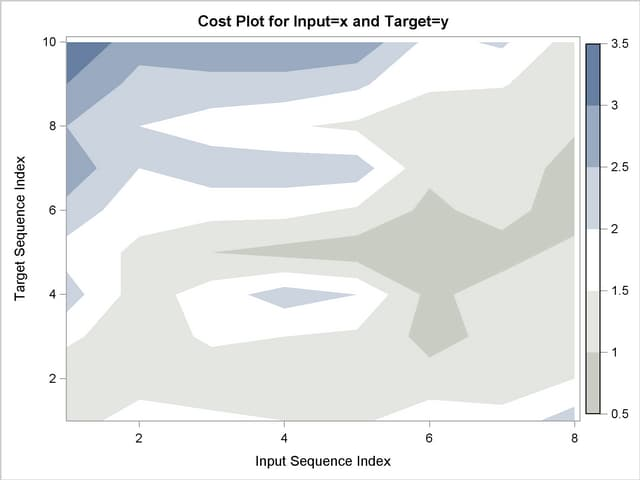Output 22.2.15 Cost Statistics
Cost Statistics
Cost Number Total Average Standard Deviation Minimum Maximum Input Mean Target Mean Minimum Path Mean Maximum Path Mean
Absolute 12 15.00000 1.250000 1.138180 0 3.000000 1.875000 1.500000 1.875000 0.8823529
Relative 12 2.25844 0.188203 0.160922 0 0.500000 0.282305 0.225844 0.282305 0.1328495

Relative Costs based on Target Sequence values

Output 22.2.16 Time Warp Plot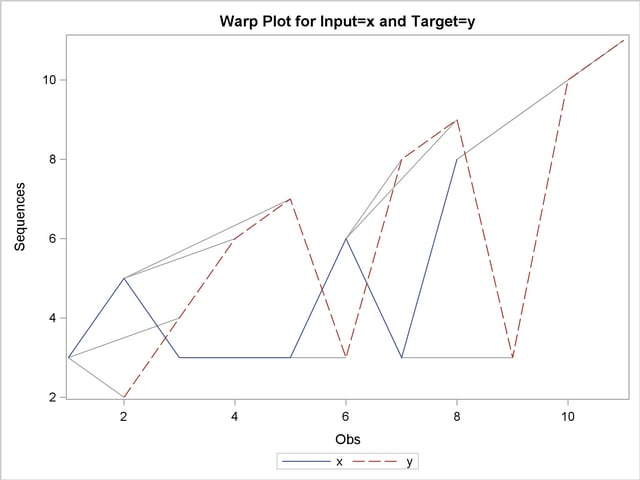Output 22.2.17 Time Warp Scaled Plot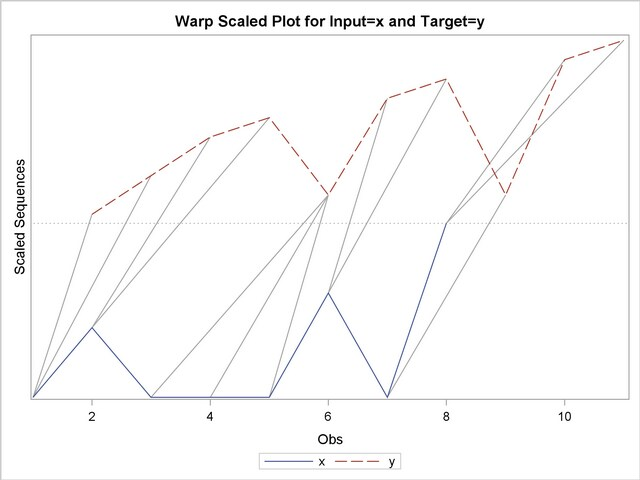The following statements repeat the above similarity analysis on the example data set with warping limits:

```   ods graphics on;
proc similarity data=test out=_null_
print=all plot=all;
input x;
target y / measure=absdev
compress=(localabs=2)
expand=(localabs=2);
run;
```

The COMPRESS=(LOCALABS=2) option limits local absolute compression to 2. The EXPAND=(LOCALABS=2) option limits local absolute expansion to 2.

Output 22.2.18 Path Plot with Warping Limits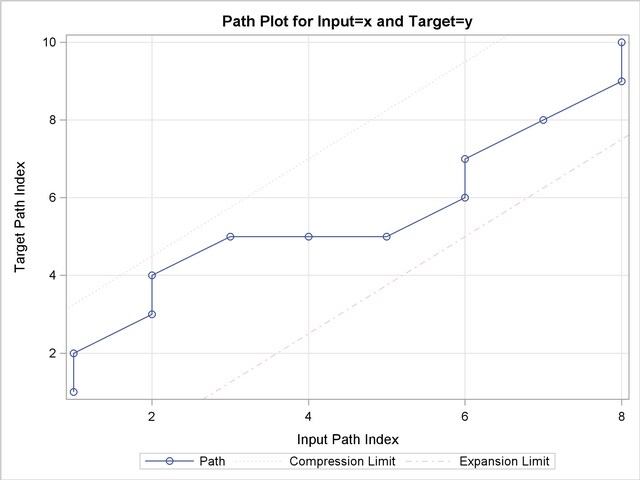Output 22.2.19 Warped Path Limits
Path Limits
Limit Specified
Absolute
Specified Percentage Minimum Allowed Maximum Allowed Applied
Compression 2 None 2 9 2
Expansion 2 None 0 7 2

Output 22.2.20 Cost Plot with Warping Limits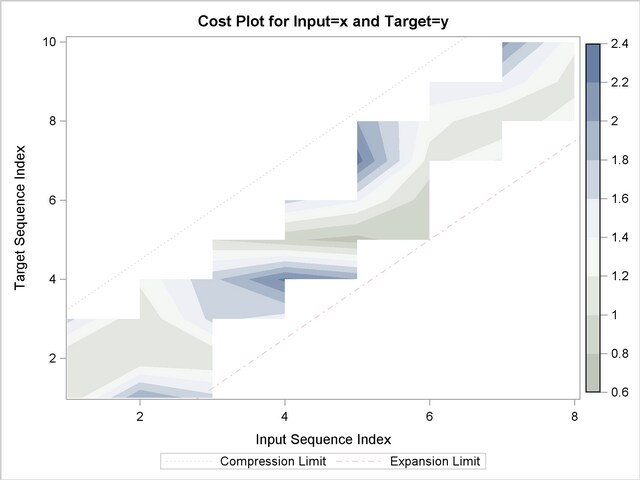The following statements repeat the above similarity analysis on the example data set but store the results in output data sets:

```   proc similarity data=test out=series
outsequence=sequences outpath=path outsum=summary;
input x;
target y / measure=absdev
compress=(localabs=2)
expand=(localabs=2);
run;
```

The OUT=SERIES, OUTSEQUENCE=SEQUENCES, OUTPATH=PATH, and OUTSUM=SUMMARY options specify that the output time series, time sequences, path analysis, and summary data sets be created, respectively.

Note: This procedure is experimental.Previous Page | Next Page | Top of Page